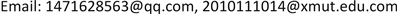1. 引言

2. 相关研究综述2.1. 江苏省5A级景区概述

2.2. 江苏省5A级景区的数据信息

Tickets and accommodation fees for 5A scenic spots in Jiangsu Provinc

The distance between some scenic spot
km1234567891011121314151617181920212223
10
2530
31011580
45685884720
54554693882220
63133492582291400
72172672733992691880
8164200753972453190
915920678464413284299700
1056110454954132842351301020
11177213103365314186209771091420
121111251584833642721292132201471850
13169216137397290181991802121391131220
1420524213233928515622310613817140171840
1522025613534433520528082109185872551851050
169614348516435307257110101281591691681841910
1760108514994162872571331145144139143170189350
189113824448381253266105120579214112711813775600
1921024713734033220027186113176782451761009.61951821270
2032601075344493202841711675818314017620822810456982220
211142114529446318262169164701801181732052251016696216350
222818129545474333273184180851991261922242401178211123135280
23518598506423294236163179551779215018721910957932106656700

3. 旅游路线的数学模型规划3.1. TSP模型问题

TSP问题  从描述上来看是在给定n个城市和各城市之间的距离的条件下，寻找一条遍历所有城市的最短路径，并且保证每个城市只被访问一次。针对江苏省的5A级景区的旅行路线规划，它的要求主要有：1、每一个景区仅游览一次；2、不含子巡回；3、整个旅游费最合理，4、旅游路线最短。

3.2. 模型假设

1) 假设任意两个风景区都有便捷的公路；

2) 不考虑浏览风景区的先后顺序；

3) 假设旅游费用不包括餐饮，不考虑其他省到达江苏省的费用；

4) 只考虑经济酒店，不考虑节假日带来的单位住宿费的提高；

5) 不区分高速公路和普通公路；

6) 假设在旅途中只有高铁或自驾两种交通工具，公交车的计费方式为路程乘以单位耗油费，并且在旅途中尽量以乘坐高铁为主，公交车为辅。

3.3. 符号说明

1) s c e n e = { 1 , 2 , ⋯ , 23 } 表示风景区集合，其中1代指出发地；

2) d i j 表示风景区和风景区两地之间的距离；

3) p i j 为二值变量，表示路线中风景区j紧随取值1，否则为0；

4) PZ是乘坐过公交车程中的费用；

5) PG是旅游过程中乘坐高铁的票价；

6) Q i d i a n i 乘坐高铁的起始位置；

7) Z h o n g d i a n i 乘坐高铁的终点位置；

8) P n 是公路上行驶的单位里程耗油费(0.6672元/公里)；

9) PL是对应城市等级的单位住宿费；

10) PM是对应旅游景区的门票费用；

11) N L i , j 对应城市的住宿费次数；

12) D T i , j 是第i次旅游路线中在普通公路或高数公路上行驶的里程；

3.4. 模型建立3.4.1. 目标函数一：最短路径

min z = ∑ i n ∑ j n d i j p i j ,     s .t . (3.1)

∀ j ∈ s c e n e : ∑ i ∈ s c e n e p i j = 1 (3.2)

∀ i ∈ s c e n e : ∑ i ∈ s c e n e p i j = 1 (3.3)

∀ i , j ∈ s c e n e : p i j ∈ { 0 , 1 } (3.4)

v i − v j + n p ≤ n − 1 , v i , v j ≥ 0 , i = 1 , 2 , ⋯ , n , j = 2 , 3 , ⋯ , n 且 i ≠ j (3.5)

v i ≤ n − 1 , v i 取 整 数 (3.6)

v 4 − v 5 + n ≤ n − 1

v 5 − v 4 + n ≤ n − 1

3.4.2. 目标函数二：预算费用

P 总 = ∑ i = 1 3 P i (3.7)

P 1 = ∑ i , j ∈ s c e n e n P G ( Q i d i a n i , Z h o n g d i a n j ) (3.8)

P 2 = ∑ i , j n d i j × P n (3.9)

P 3 = ∑ i ∈ s c e n e P M + ∑ j ∈ s c e n e P L × N L i , j (3.10)

4. 蚂蚁算法解决TSP模型4.1. 蚁群算法原理

1) α i j ( t ) 表示在t时刻，景区i到景区j间路径的信息强度。

2) β i j ( t ) 表示景区i和景区j的启发信息。一般情况下 β i j ( t ) = 1 d i j 。

3) p i j s ( t ) 表示在t时刻，蚂蚁s由景区i转移至景区j的概率。

p i j s ( t ) = { α i j k ( t ) β i j l ( t ) ∑ r ∈ T a b l e s α i r k ( t ) β i r l ( t ) j ∈ T a b l e s 0 否 则

r = { arg max { [ α ( i , j ) ] [ β ( i , j ) ] l }             若 q ≤ q 0 R                                                                                           否 则

α i j ( t + 1 ) = ( 1 − p ) ⋅ α i j ( t ) + Δ α i j

Δ α i j = ∑ k = 1 m Δ α i j k

Δ α i j k = { Q L k ,     如 果 蚂 蚁   k   在   t   时 刻 到 t + 1 时 刻 通 过 i , j 0 ,                                                         否 则

4.2. 蚁群算法的实现步骤

1) m c ← 0 各 α i j 和 Δ α i j 的初始化；将n只蚂蚁至于m个点上。

2) 将各蚂蚁的初始出发点置于当前解集中；对于每只蚂蚁k，按概率 p i j k 移至下一项点j，将顶点j置于当前解集。

3) 计算各蚂蚁的路径长度，记录当前的最好解。

4) 按更新方程修改轨迹强度。

5) 对各边弧 ( i , j ) ，置 Δ τ i j ← 0 ， n c ← n c + 1 。

6) 若小于预定的迭代次数且无退化行为，则转至步骤(2)。

7) 输出目前最好解。

4.3. 改进蚁群算法的求解步骤

1) 初始化：迭代步数nc设置为0，在从大量的路径中选择比较优的路径，在这些路径留下信息素。将m只蚂蚁置于n个顶点上。

2) 根据当前位置调节各个值。

3) 将各蚂蚁的初始出发点置于当前解集中；对每只蚂蚁 k ( k = 1 , 2 , ⋯ , m ) ，按照概率移至下一顶点j；将顶点j置于当前解集。

4) 对每只蚂蚁进行交叉变异操作，找出全局极值和全局极值位置。

5) 计算各蚂蚁的路径长度；记录当前的最好解。

6) 按更新方程修改轨迹强度。

7) 对各边弧 ( i , j ) ，置 Δ τ i j ← 0 ， n c ← n c + 1 。

8) 若迭代步数小于预定的迭代次数且无退化行为(即找到的都是相同的解)，则转至步骤(2)。

9) 输出目前最好解。

4.3.1. 改进的蚁群算法的运行结果和分析

14 → 11 → 19 → 15 → 8 → 9 → 18 → 3 → 17 → 10 → 16 → 20 → 22 → 2 → 21 → 1 → 23 → 12 → 13 → 7 → 6 → 5 → 4。

4.3.2. 模型总结

5. 总结